Updating search results...

# 31 Results

View
Selected filters:
• dlc-blended-learning-math-3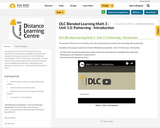Conditional Remix & Share Permitted
CC BY-NC-SA
Rating
0.0 stars

The purpose of this lesson is to introduce the unit on patterning and activate prior knowledge about patterning.

Included are the support materials for Grade 2 Blended Learning Math - Unit 1.0: Patterning - Introduction:

Subject:
Math
Material Type:
Activity/Lab
Homework/Assignment
Lesson
Provider:
Sun West Distance Learning Centre (DLC)
04/04/2019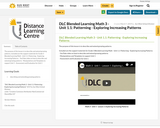Conditional Remix & Share Permitted
CC BY-NC-SA
Rating
0.0 stars

The purpose of this lesson is to describe and extend growing patterns.

Included are the support materials for Grade 3 Blended Learning Math - Unit 1.1: Patterning - Exploring Increasing Patterns:
- YouTube video on how to describe and extend growing patterns

Subject:
Math
Material Type:
Activity/Lab
Homework/Assignment
Lesson
Provider:
Sun West Distance Learning Centre (DLC)
04/04/2019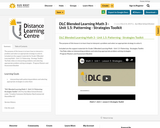Conditional Remix & Share Permitted
CC BY-NC-SA
Rating
0.0 stars

The purpose of this lesson is to learn how to interpret a problem and select an appropriate strategy to solve it.

Included are the support materials for Grade 3 Blended Learning Math - Unit 1.5: Patterning - Strategy Toolkit:
- YouTube video on interpreting problems and selecting appropriate problem solving strategies

Subject:
Math
Material Type:
Activity/Lab
Homework/Assignment
Lesson
Provider:
Sun West Distance Learning Centre (DLC)
04/04/2019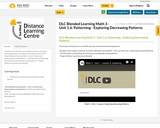Conditional Remix & Share Permitted
CC BY-NC-SA
Rating
0.0 stars

The purpose of this lesson is to identify, describe and extend decreasing patterns.

Included are the support materials for Grade 3 Blended Learning Math - Unit 1.6: Patterning - Exploring Decreasing Patterns:
- YouTube video on identifying, describing and extending decreasing patterns

Subject:
Math
Material Type:
Activity/Lab
Homework/Assignment
Lesson
Provider:
Sun West Distance Learning Centre (DLC)
04/04/2019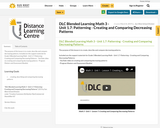Conditional Remix & Share Permitted
CC BY-NC-SA
Rating
0.0 stars

The purpose of this lesson is to create, describe and compare decreasing patterns.

Included are the support materials for Grade 3 Blended Learning Math - Unit 1.7: Patterning - Creating and Comparing Decreasing Patterns:
- YouTube video on creating and comparing decreasing patterns

Subject:
Math
Material Type:
Activity/Lab
Homework/Assignment
Lesson
Provider:
Sun West Distance Learning Centre (DLC)
04/04/2019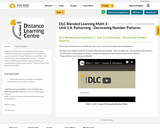Conditional Remix & Share Permitted
CC BY-NC-SA
Rating
0.0 stars

The purpose of this lesson is to identify, describe, create, extend and compare decreasing patterns.

Included are the support materials for Grade 3 Blended Learning Math - Unit 1.8: Patterning - Decreasing Number Patterns:
- YouTube video on identifying, describing, creating, extending and comparing decreasing number patterns

Subject:
Math
Material Type:
Activity/Lab
Homework/Assignment
Lesson
Provider:
Sun West Distance Learning Centre (DLC)
04/04/2019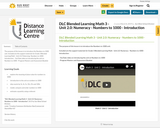Conditional Remix & Share Permitted
CC BY-NC-SA
Rating
0.0 stars

The purpose of this lesson is to introduce the Numbers to 1000 unit.

Included are the support materials for Grade 3 Blended Learning Math - Unit 2.0: Patterning -Numbers to 1000 - Introduction:
- YouTube video on introducing the unit on Numbers to 1000

Subject:
Math
Material Type:
Activity/Lab
Homework/Assignment
Lesson
Provider:
Sun West Distance Learning Centre (DLC)
04/04/2019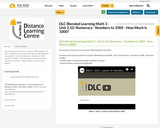Conditional Remix & Share Permitted
CC BY-NC-SA
Rating
0.0 stars

The purpose of this lesson is to represent 1000 using Base Ten blocks.

Included are the support materials for Grade 3 Blended Learning Math - Unit 2.10: Numeracy - Numbers to 1000 - How Much is 1000?:
- YouTube video on representing 1000 using Base Ten blocks

Subject:
Math
Material Type:
Activity/Lab
Homework/Assignment
Lesson
Provider:
Sun West Distance Learning Centre (DLC)
04/04/2019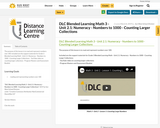Conditional Remix & Share Permitted
CC BY-NC-SA
Rating
0.0 stars

The purpose of this lesson is to read and represent numbers over 100.

Included are the support materials for Grade 3 Blended Learning Math - Unit 2.1: Numeracy - Numbers to 1000 - Counting Larger Collections:
- YouTube video on counting larger collections

Subject:
Math
Material Type:
Activity/Lab
Homework/Assignment
Lesson
Provider:
Sun West Distance Learning Centre (DLC)
04/04/2019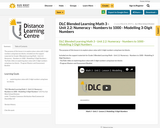Conditional Remix & Share Permitted
CC BY-NC-SA
Rating
0.0 stars

The purpose of this lesson is to explore place value with 3-digit numbers using base ten blocks.

Included are the support materials for Grade 3 Blended Learning Math - Unit 2.2: Numeracy - Numbers to 1000 - Modelling 3-Digit Numbers:
- YouTube video on exploring place value with 3-digit numbers using base ten blocks

Subject:
Math
Material Type:
Activity/Lab
Homework/Assignment
Lesson
Provider:
Sun West Distance Learning Centre (DLC)
04/04/2019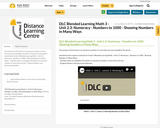Conditional Remix & Share Permitted
CC BY-NC-SA
Rating
0.0 stars

The purpose of this lesson is to represent numbers in more than one way using Base Ten blocks.

Included are the support materials for Grade 3 Blended Learning Math - Unit 2.3: Numeracy - Numbers to 1000 - Showing Numbers in Many Ways:
- YouTube video on using Base Ten blocks to represent numbers in more than one way

Subject:
Math
Material Type:
Activity/Lab
Homework/Assignment
Lesson
Provider:
Sun West Distance Learning Centre (DLC)
04/04/2019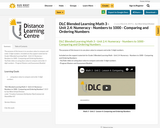Conditional Remix & Share Permitted
CC BY-NC-SA
Rating
0.0 stars

The purpose of this lesson is to use place value to compare and order 3-digit numbers.

Included are the support materials for Grade 3 Blended Learning Math - Unit 2.4: Numeracy - Numbers to 1000 - Comparing and Ordering Numbers:
- YouTube video on using place value to compare and order 3-digit numbers

Subject:
Math
Material Type:
Activity/Lab
Homework/Assignment
Lesson
Provider:
Sun West Distance Learning Centre (DLC)
04/04/2019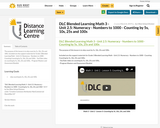Conditional Remix & Share Permitted
CC BY-NC-SA
Rating
0.0 stars

The purpose of this lesson is to skip count by 5s, 10s, 25s and 100s.

Included are the support materials for Grade 3 Blended Learning Math - Unit 2.5: Numeracy - Numbers to 1000 - Counting Counting by 5s, 10s, 25s and 100s:
- YouTube video on counting by 5s, 10s, 25s and 100s,

Subject:
Math
Material Type:
Activity/Lab
Homework/Assignment
Lesson
Provider:
Sun West Distance Learning Centre (DLC)
04/04/2019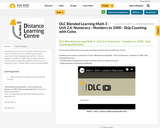Conditional Remix & Share Permitted
CC BY-NC-SA
Rating
0.0 stars

The purpose of this lesson is to use skip counting to find the value of a collection of coins.

Included are the support materials for Grade 3 Blended Learning Math - Unit 2.6: Numeracy - Numbers to 1000 - Skip Counting with Coins:
- YouTube video on using skip counting to find the value of a collection of coins

Subject:
Math
Material Type:
Activity/Lab
Homework/Assignment
Lesson
Provider:
Sun West Distance Learning Centre (DLC)
04/04/2019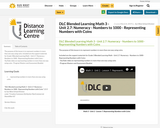Conditional Remix & Share Permitted
CC BY-NC-SA
Rating
0.0 stars

The purpose of this lesson is to represent numbers in more than one way using coins.

Included are the support materials for Grade 3 Blended Learning Math - Unit 2.7: Numeracy - Numbers to 1000 - Representing Numbers with Coins:
- YouTube video on representing numbers in more than one way using coins

Subject:
Math
Material Type:
Activity/Lab
Homework/Assignment
Lesson
Provider:
Sun West Distance Learning Centre (DLC)
04/04/2019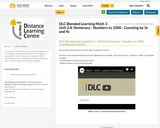Conditional Remix & Share Permitted
CC BY-NC-SA
Rating
0.0 stars

The purpose of this lesson is to practice skip counting by 3s and 4s.

Included are the support materials for Grade 3 Blended Learning Math - Unit 2.8: Numeracy - Numbers to 1000 - Counting by 3s and 4s:
- YouTube video on skip counting by 3s and 4s

Subject:
Math
Material Type:
Activity/Lab
Homework/Assignment
Lesson
Provider:
Sun West Distance Learning Centre (DLC)
04/04/2019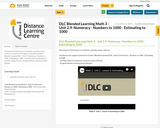Conditional Remix & Share Permitted
CC BY-NC-SA
Rating
0.0 stars

The purpose of this lesson is to estimate a quantity using a referent.

Included are the support materials for Grade 3 Blended Learning Math - Unit 2.9: Numeracy - Numbers to 1000 - Estimating to 1000:

Subject:
Math
Material Type:
Activity/Lab
Homework/Assignment
Lesson
Provider:
Sun West Distance Learning Centre (DLC)
04/04/2019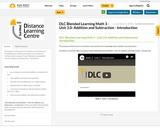Conditional Remix & Share Permitted
CC BY-NC-SA
Rating
0.0 stars

The purpose of this lesson is to introduce and activate prior knowledge about addition and subtraction.

Included is a YouTube video to support Grade 3 Blended Learning Math - Unit 3.0: Addition and Subtraction - Introduction

Subject:
Math
Material Type:
Activity/Lab
Homework/Assignment
Lesson
Provider:
Sun West Distance Learning Centre (DLC)
03/14/2019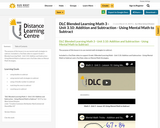Conditional Remix & Share Permitted
CC BY-NC-SA
Rating
0.0 stars

The purpose of this lesson is to use mental math strategies to subtract.

Included is a YouTube video to support Grade 3 Blended Learning Math - Unit 3.10: Addition and Subtraction - Using Mental Math to Subtract and a YouTube video on Mental Math Strategies.

Subject:
Math
Material Type:
Activity/Lab
Homework/Assignment
Lesson
Provider:
Sun West Distance Learning Centre (DLC)
03/14/2019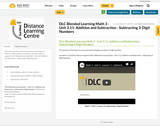Conditional Remix & Share Permitted
CC BY-NC-SA
Rating
0.0 stars

The purpose of this lesson is to use personal strategies to subtract 3-digit numbers.

Included is a YouTube video to support Grade 3 Blended Learning Math - Unit 3.11: Addition and Subtraction - Subtracting 3-Digit Numbers

Subject:
Math
Material Type:
Activity/Lab
Homework/Assignment
Lesson
Provider:
Sun West Distance Learning Centre (DLC)